CA-CPT Question Paper December - 2015 (Session 2)

# CA-CPT Question Paper December - 2015 (Session 2)

Test Description

## 100 Questions MCQ Test | CA-CPT Question Paper December - 2015 (Session 2)

CA-CPT Question Paper December - 2015 (Session 2) for CA CPT 2022 is part of CA CPT preparation. The CA-CPT Question Paper December - 2015 (Session 2) questions and answers have been prepared according to the CA CPT exam syllabus.The CA-CPT Question Paper December - 2015 (Session 2) MCQs are made for CA CPT 2022 Exam. Find important definitions, questions, notes, meanings, examples, exercises, MCQs and online tests for CA-CPT Question Paper December - 2015 (Session 2) below.
Solutions of CA-CPT Question Paper December - 2015 (Session 2) questions in English are available as part of our course for CA CPT & CA-CPT Question Paper December - 2015 (Session 2) solutions in Hindi for CA CPT course. Download more important topics, notes, lectures and mock test series for CA CPT Exam by signing up for free. Attempt CA-CPT Question Paper December - 2015 (Session 2) | 100 questions in 120 minutes | Mock test for CA CPT preparation | Free important questions MCQ to study for CA CPT Exam | Download free PDF with solutions
 1 Crore+ students have signed up on EduRev. Have you?
CA-CPT Question Paper December - 2015 (Session 2) - Question 1

### Balance of Current A/c includes

CA-CPT Question Paper December - 2015 (Session 2) - Question 2

### Cobb – douglas production function studies about:

CA-CPT Question Paper December - 2015 (Session 2) - Question 3

### Macro Economics is also called as ______ economics.

CA-CPT Question Paper December - 2015 (Session 2) - Question 4

If the marginal (additional) opportunity cost is a constant, then the PPC would be a ______

CA-CPT Question Paper December - 2015 (Session 2) - Question 5

A firm’s average total cost is Rs. 300 at 5 Units of output and Rs.320 at 6 units of output. The marginal cost of producing the 6th unit is:

CA-CPT Question Paper December - 2015 (Session 2) - Question 6

The kinked demand curve model of oligopoly assumes that

CA-CPT Question Paper December - 2015 (Session 2) - Question 7

In case of perfect substitutes, Cross elasticity of demand is ____

CA-CPT Question Paper December - 2015 (Session 2) - Question 8

A firm producing 7 units of output has an average total cost of Rs. 150 and has to pay Rs.350 to its fixed factors of production whether it produces or not. How much of the average total cost is made up of variable costs.

CA-CPT Question Paper December - 2015 (Session 2) - Question 9

Which of the following equations of cost is incorrect?

CA-CPT Question Paper December - 2015 (Session 2) - Question 10

Match the following: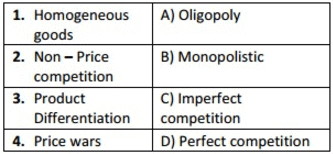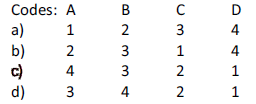CA-CPT Question Paper December - 2015 (Session 2) - Question 11

A competitive firm in the short run incur losses. The firm continues production, if:

CA-CPT Question Paper December - 2015 (Session 2) - Question 12

Product heterogeneity is a common feature in

CA-CPT Question Paper December - 2015 (Session 2) - Question 13

Which of the following is related to Law of variable proportions is correct?

CA-CPT Question Paper December - 2015 (Session 2) - Question 14

Supply curve in case of perfectly Elastic:

CA-CPT Question Paper December - 2015 (Session 2) - Question 15

Which one of the following is not correct for deductive method:

CA-CPT Question Paper December - 2015 (Session 2) - Question 16

At the best level of output the condition under monopolist is _____

CA-CPT Question Paper December - 2015 (Session 2) - Question 17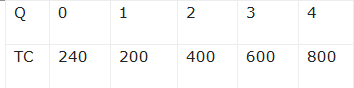AVC at 2nd unit

CA-CPT Question Paper December - 2015 (Session 2) - Question 18

AFC is 20 at 4 units of output what will be it at 8 units of output

CA-CPT Question Paper December - 2015 (Session 2) - Question 19

If Rs.1,000 of variable cost at 4 units and fixed cost is Rs.400. Then find out AC at 4 units?

CA-CPT Question Paper December - 2015 (Session 2) - Question 20

On the basis of the due date given below what will be the volume of budget deficit

a) Revenue receipts Rs.5,72,811 crores
b) Capital receipts Rs.4,51,676 crores

• i. Loan recovered other receipts Rs.33,194 crores
• ii. Borrowing & other liability Rs.4,18,482 crores

c) Revenue expenditure Rs.9,11,809 crores
d) Capital expenditure Rs.1,12,678 crores

CA-CPT Question Paper December - 2015 (Session 2) - Question 21

Which cities are involved in developing Golden Quadrilateral corridor under national highways of development project.

CA-CPT Question Paper December - 2015 (Session 2) - Question 22

Which of the following is recasted as AAJEEVIKA

CA-CPT Question Paper December - 2015 (Session 2) - Question 23

Fishing and Logging are included in which sector?

CA-CPT Question Paper December - 2015 (Session 2) - Question 24

NLM was recasted into ____ in 2009

CA-CPT Question Paper December - 2015 (Session 2) - Question 25

Suppose a shopkeeper buys inputs worth Rs.50,000 and his sales are worth Rs.1,00,000 in a month. The input tax rate is 8% and output tax rate is 20%. What is Value added tax here after set off of input tax credit?

CA-CPT Question Paper December - 2015 (Session 2) - Question 26

According to traditional approach money serves as

CA-CPT Question Paper December - 2015 (Session 2) - Question 27

According to RBI (1998) modified new monetary aggregates as:

CA-CPT Question Paper December - 2015 (Session 2) - Question 28

Which of the following is not a function of IMF

CA-CPT Question Paper December - 2015 (Session 2) - Question 29

Farm credit package was introduced in the year

CA-CPT Question Paper December - 2015 (Session 2) - Question 30

Sex Ratio is favourable to Women in which State?

CA-CPT Question Paper December - 2015 (Session 2) - Question 31

National Manufacturing policy was introduced in the year_____

CA-CPT Question Paper December - 2015 (Session 2) - Question 32

Which of the following is not a method of privatization?

CA-CPT Question Paper December - 2015 (Session 2) - Question 33

Which of the following is used to control inflation?

CA-CPT Question Paper December - 2015 (Session 2) - Question 34

Which of the following is not a main objective of Nationalization of Commercial Banks

CA-CPT Question Paper December - 2015 (Session 2) - Question 35

Which of the following statements is incorrect?

CA-CPT Question Paper December - 2015 (Session 2) - Question 36

Mark the incorrect statement

CA-CPT Question Paper December - 2015 (Session 2) - Question 37

Capital Account Convertibility is done by_____

CA-CPT Question Paper December - 2015 (Session 2) - Question 38

Which of the following committee modified direct and indirect taxes?

CA-CPT Question Paper December - 2015 (Session 2) - Question 39

Which of the following is related to Dependency Ratio?

CA-CPT Question Paper December - 2015 (Session 2) - Question 40

Personal Disposable income is equal to Personal income

CA-CPT Question Paper December - 2015 (Session 2) - Question 41

Plant Load Factor is used to measure ______

CA-CPT Question Paper December - 2015 (Session 2) - Question 42

Janani Suraksha Yojana was started with an aim to____

CA-CPT Question Paper December - 2015 (Session 2) - Question 43

The co-existence of raising prices and fall in demand leading to unemployment is called __

CA-CPT Question Paper December - 2015 (Session 2) - Question 44

IFSC is _____ digit alphanumeric code which is an unique for each branch of a bank

CA-CPT Question Paper December - 2015 (Session 2) - Question 45

If National domestic capital at factor cost is Rs.4635 crores, subsidies are Rs.50 crores, Factor income from abroad is Rs.40 crores, Factor income paid is Rs.50 crores, Depreciation is Rs.200 crores then National Income is ____

CA-CPT Question Paper December - 2015 (Session 2) - Question 46

All of the following developments were noticed during 1991 (when economic reforms were enforced) except one. Identify it.

CA-CPT Question Paper December - 2015 (Session 2) - Question 47

In persuasion to prevent and regulate the credit of commercial banks by Central Bank, which of the following is followed?

CA-CPT Question Paper December - 2015 (Session 2) - Question 48

At shut down point :

CA-CPT Question Paper December - 2015 (Session 2) - Question 49

If as a result of change in price, the quantity supplied of the good remains unchanged, we
say elasticity of supply is:

CA-CPT Question Paper December - 2015 (Session 2) - Question 50

The conditions of long-period equilibrium for the firm operative under perfect competition
are:
(1) MC = MR
(2) AR = MR
(3) AC = AR
(4) AC = MC

CA-CPT Question Paper December - 2015 (Session 2) - Question 51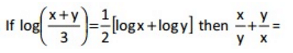CA-CPT Question Paper December - 2015 (Session 2) - Question 52

If a2 + b2 = 7ab then log(a + b) =

CA-CPT Question Paper December - 2015 (Session 2) - Question 53

U = {x/x is a positive integer less than 15}.

A = {2,8,10,14} B = {3,6,8,14} then

CA-CPT Question Paper December - 2015 (Session 2) - Question 54

if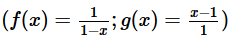then gof(x) =

CA-CPT Question Paper December - 2015 (Session 2) - Question 55

If a, b, c are in G.P & x, y be AM’s between a, b & b, c respectively then a/x + c/y =

CA-CPT Question Paper December - 2015 (Session 2) - Question 56

The first terms of two G.Ps are equal and 3rd term of Ist GP and 5th term of second GP are equal then the ratio of 67th term of Ist GP to 133 rd term of 2nd GP is ____

CA-CPT Question Paper December - 2015 (Session 2) - Question 57

A man earns 10,000 P.M. His salary increases by 200/- per year. What is his total salary in 5 years?

CA-CPT Question Paper December - 2015 (Session 2) - Question 58

The length of the median of D ABC through the vertex B is ___ if the vertices are A(1,2); B(2, -1); C(3,4)

CA-CPT Question Paper December - 2015 (Session 2) - Question 59

The graph to express the inequality y ≥ 3x is

CA-CPT Question Paper December - 2015 (Session 2) - Question 60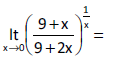CA-CPT Question Paper December - 2015 (Session 2) - Question 61

If the sum of the roots of quadratic equation is ‘3’ and the sum of the cubes of the roots is ‘7’ then the equation is

CA-CPT Question Paper December - 2015 (Session 2) - Question 62

If the difference between the corresponding roots of the equations x2 + ax + b = 0 and x2 + bx + a = 0 is same and a ≠ b then

CA-CPT Question Paper December - 2015 (Session 2) - Question 63

if y =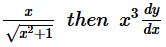=

CA-CPT Question Paper December - 2015 (Session 2) - Question 64

If Y = a. emx + b e –mx then d2y/dx2 =

CA-CPT Question Paper December - 2015 (Session 2) - Question 65

If the curve Y2 = AX4 + B passes through the point P(1,2). The value of dx/ dy at P is 4. Then

CA-CPT Question Paper December - 2015 (Session 2) - Question 66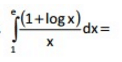CA-CPT Question Paper December - 2015 (Session 2) - Question 67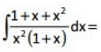CA-CPT Question Paper December - 2015 (Session 2) - Question 68

How many permutations can be formed from the letters of the word “PARALLEL” in which 3L’s do not come together?

CA-CPT Question Paper December - 2015 (Session 2) - Question 69

In how many ways that 8 members including the President & Vice president can be arranged at a round table so that the president and Vice president do not come together is ___

CA-CPT Question Paper December - 2015 (Session 2) - Question 70

How many numbers can be formed using the digits 2,3,4,1,6 that are greater than 1000 is _

CA-CPT Question Paper December - 2015 (Session 2) - Question 71

X varies as cube of Y & Y varies as 5th root of Z and X varies as nth power of ‘Z’ then n =

CA-CPT Question Paper December - 2015 (Session 2) - Question 72

Instead of walking along two adjacent sides of a rectangular field, a boy took short cut along the diagonal and saved a distance equal to the half of the longer side. Then the ratio of shorter side of the rectangular field to its longer side is _______

CA-CPT Question Paper December - 2015 (Session 2) - Question 73

If the ratio of two numbers is 3: 5. If 9 is subtracted from each then the ratio becomes 12: 23. Then the largest number is ___

CA-CPT Question Paper December - 2015 (Session 2) - Question 74

Rs.3,000 is payable at the end of each year at the rate of 9% compounded annually for 3yrs then the amount of annuity will be:

CA-CPT Question Paper December - 2015 (Session 2) - Question 75

Useful life of a machine is estimated to be 10 years and the cost of asset is Rs.10,000. If the rate of depreciation is 10% p.a., then the scrap value is ____

CA-CPT Question Paper December - 2015 (Session 2) - Question 76

For two events A, B which of the following is true?

CA-CPT Question Paper December - 2015 (Session 2) - Question 77

The probability of getting atleast 3 heads in 8 tosses of an unbiased coin is ______

CA-CPT Question Paper December - 2015 (Session 2) - Question 78

If 7:6 are the odds favour for the person ‘A’ will alive 5 years hence and 5: 3 are the odds favour for the person ‘B’ will alive 5years hence, then the probability for atleast one will alive 5 years hence is

CA-CPT Question Paper December - 2015 (Session 2) - Question 79

Mean of 50 observations is same as that of mean of observations after an observation 45 is dropped. What is the mean of 50 observations?

CA-CPT Question Paper December - 2015 (Session 2) - Question 80

Let X takes the values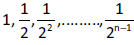and the GM of X is 1/2k  then the k value is

CA-CPT Question Paper December - 2015 (Session 2) - Question 81

The standard deviation of two numbers is

CA-CPT Question Paper December - 2015 (Session 2) - Question 82

Which of the following is least affected by extreme values?

CA-CPT Question Paper December - 2015 (Session 2) - Question 83

If the standard deviation of X is 9 then V(10+2X) =?

CA-CPT Question Paper December - 2015 (Session 2) - Question 84

The Spearman’s rank correlation coefficient is 0.95 and Σd2 =4, find the number of pairs of observations

CA-CPT Question Paper December - 2015 (Session 2) - Question 85

If the two regression lines are perpendicular to each other then

CA-CPT Question Paper December - 2015 (Session 2) - Question 86

The AM of Laspeyre’s and Paasche’s indices is_____ index number.

CA-CPT Question Paper December - 2015 (Session 2) - Question 87

Shifted price index =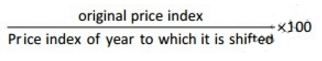CA-CPT Question Paper December - 2015 (Session 2) - Question 88

The headings of the rows given in the first column of a table are called

CA-CPT Question Paper December - 2015 (Session 2) - Question 89

The relationship between two variables are shown in

CA-CPT Question Paper December - 2015 (Session 2) - Question 90

Mode can be obtained from____

CA-CPT Question Paper December - 2015 (Session 2) - Question 91

Data taken from the publication “Agricultural situation in India” will be considered as

CA-CPT Question Paper December - 2015 (Session 2) - Question 92

285 is summation of 3 numbers. Ratio between 2nd and 3rd numbers is 6:5. Ratio between 1st and 2nd numbers is 3:7. The 3rd number is?

CA-CPT Question Paper December - 2015 (Session 2) - Question 93

If X ~ B (n,p) then the distribution of Y=n-X follows

CA-CPT Question Paper December - 2015 (Session 2) - Question 94

If X ~ B (6,1/3) and k P(X=4) = P(X=2) then the value of k is

CA-CPT Question Paper December - 2015 (Session 2) - Question 95

In a Poisson distribution if P(X=1) = P(X = 2) the standard deviation is

CA-CPT Question Paper December - 2015 (Session 2) - Question 96

If X ~ N( µ , σ2) , then which of the following is true

CA-CPT Question Paper December - 2015 (Session 2) - Question 97

The number of possible random samples without replacement drawn from a population is

CA-CPT Question Paper December - 2015 (Session 2) - Question 98

If n units are selected from N population units, the sampling fraction is given as

CA-CPT Question Paper December - 2015 (Session 2) - Question 99

If X ~ F (m,n) then E (X)=

CA-CPT Question Paper December - 2015 (Session 2) - Question 100

If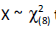then SD (X)=

 Use Code STAYHOME200 and get INR 200 additional OFF Use Coupon Code
Information about CA-CPT Question Paper December - 2015 (Session 2) Page
In this test you can find the Exam questions for CA-CPT Question Paper December - 2015 (Session 2) solved & explained in the simplest way possible. Besides giving Questions and answers for CA-CPT Question Paper December - 2015 (Session 2), EduRev gives you an ample number of Online tests for practice